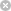RTXI  2.1fir_dsgn.cpp
Go to the documentation of this file.
1 /*
2  * Functions for class FirFilterDesign
3  */
4
5 #include <gsl/gsl_math.h>
6 #include <math.h>
7 #include <stdlib.h>
8
9 #include "misdefs.h"
10 #include "fir_dsgn.h"
11 #include "fir_resp.h"
12
13 // default constructor
14
16 {
17  return;
18 }
19
21 {
22  Num_Taps = num_taps;
23  Imp_Resp_Coeff = new double[num_taps];
24  Original_Coeff = new double[num_taps];
25 }
26
27 FirFilterDesign::FirFilterDesign(int num_taps, double* imp_resp_coeff)
28 {
29  Num_Taps = num_taps;
30  Imp_Resp_Coeff = new double[num_taps];
31  Original_Coeff = new double[num_taps];
32
33  for (int n = 0; n < num_taps; n++) {
34  Imp_Resp_Coeff[n] = imp_resp_coeff[n];
35  Original_Coeff[n] = imp_resp_coeff[n];
36  }
37  return;
38 }
39
41  double* input_coeff)
42 {
43  int last_left, first_right, n;
44  Num_Taps = num_taps;
45  Coeff_Symmetry = symmetry;
46
47  Original_Coeff = new double[num_taps];
48  Imp_Resp_Coeff = new double[num_taps];
49  Quant_Coeff = new long[num_taps];
50  if (num_taps % 2) { // odd length
51  last_left = (num_taps - 1) / 2;
52  first_right = last_left;
53  } else { // even length
54  last_left = (num_taps - 2) / 2;
55  first_right = last_left + 1;
56  }
57
58  switch (symmetry) {
59  case FIR_SYM_EVEN_LEFT:
60  for (n = 0; n <= last_left; n++) {
61  Original_Coeff[n] = input_coeff[n];
62  Imp_Resp_Coeff[n] = input_coeff[n];
63  Quant_Coeff[n] = long(Original_Coeff[n]);
64  }
65  for (n = first_right; n < num_taps; n++) {
66  Original_Coeff[n] = input_coeff[num_taps - n - 1];
67  Imp_Resp_Coeff[n] = input_coeff[num_taps - n - 1];
68  Quant_Coeff[n] = long(Original_Coeff[n]);
69  }
70  } // end of switch on symmetry
71  return;
72 }
73
74 void
76 {
77  Num_Taps = num_taps;
78  Imp_Resp_Coeff = new double[num_taps];
79  Original_Coeff = new double[num_taps];
80 }
81
82 // method to scale coefficients
83
84 void
86 {
87  int n;
88  for (n = 0; n < Num_Taps; n++) {
89  Original_Coeff[n] = scale_factor * Original_Coeff[n];
90  Imp_Resp_Coeff[n] = Original_Coeff[n];
91  }
92  return;
93 }
94
95 // scale coefficients so that magnitude response has unity gain at passband
96 // peak
97
98 void
100 {
101  int n;
102  double passband_peak;
103  FirFilterResponse* temp_response;
104
105  temp_response = new FirFilterResponse(this, 500, 0, 0, NULL);
106  passband_peak = temp_response->GetIntervalPeak(0, 499);
107  delete temp_response;
108  for (n = 0; n < Num_Taps; n++) {
109  Imp_Resp_Coeff[n] = Original_Coeff[n] / passband_peak;
110  }
111  return;
112 }
113
114 void
116 {
117  for (int n = 0; n < Num_Taps; n++) {
118  coeff[n] = Imp_Resp_Coeff[n];
119  }
120  return;
121 }
122
123 int
125 {
126  return (Num_Taps);
127 }
128
129 double*
131 {
132  // std::cout << "in fs_dsgn, Imp_Resp_Coeff = " << (void*)Imp_Resp_Coeff <<
133  // std::endl;
134  return (Imp_Resp_Coeff);
135 }
136
137 void
139 {
140  for (int n = 0; n < Num_Taps; n++) {
141  Imp_Resp_Coeff[n] *= window->GetDataWinCoeff(n);
143  }
144 }
145
146 void
147 FirFilterDesign::ExtractPolyphaseSet(double* coeff, int decim_rate, int rho)
148 {
149  double* local_coeff;
150  local_coeff = coeff;
151
152  for (int n = rho; n < Num_Taps; n += decim_rate) {
153  *local_coeff++ = Imp_Resp_Coeff[n];
154  }
155  return;
156 }
FIR_SYM_T
Definition: fir_dsgn.h:11
void ScaleCoefficients(double scale_factor)
Definition: fir_dsgn.cpp:85
double * Imp_Resp_Coeff
Definition: fir_dsgn.h:75
long * Quant_Coeff
Definition: fir_dsgn.h:77
void ApplyWindow(GenericWindow *window)
Definition: fir_dsgn.cpp:138
void NormalizeFilter(void)
Definition: fir_dsgn.cpp:99
FIR_SYM_T Coeff_Symmetry
Definition: fir_dsgn.h:78
double * GetCoefficients(void)
Definition: fir_dsgn.cpp:130
double GetDataWinCoeff(int samp_indx)
Definition: gen_win.cpp:41
int GetNumTaps(void)
Definition: fir_dsgn.cpp:124
void ExtractPolyphaseSet(double *coeff, int decim_rate, int rho)
Definition: fir_dsgn.cpp:147
double GetIntervalPeak(int beg_indx, int end_indx)
Definition: fir_resp.cpp:119
void CopyCoefficients(double *coeff)
Definition: fir_dsgn.cpp:115
void Initialize(int num_taps)
Definition: fir_dsgn.cpp:75
double * Original_Coeff
Definition: fir_dsgn.h:76